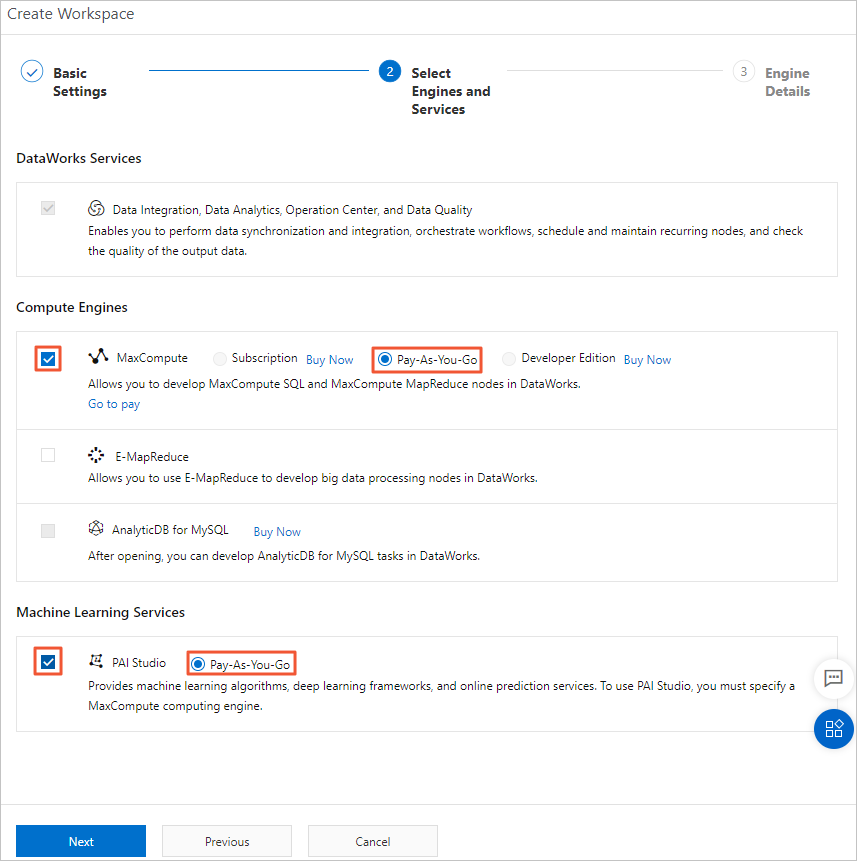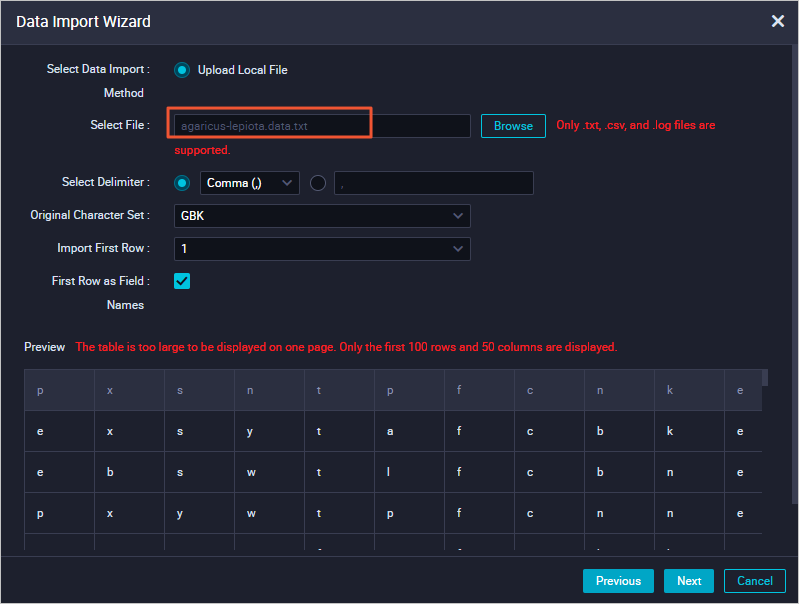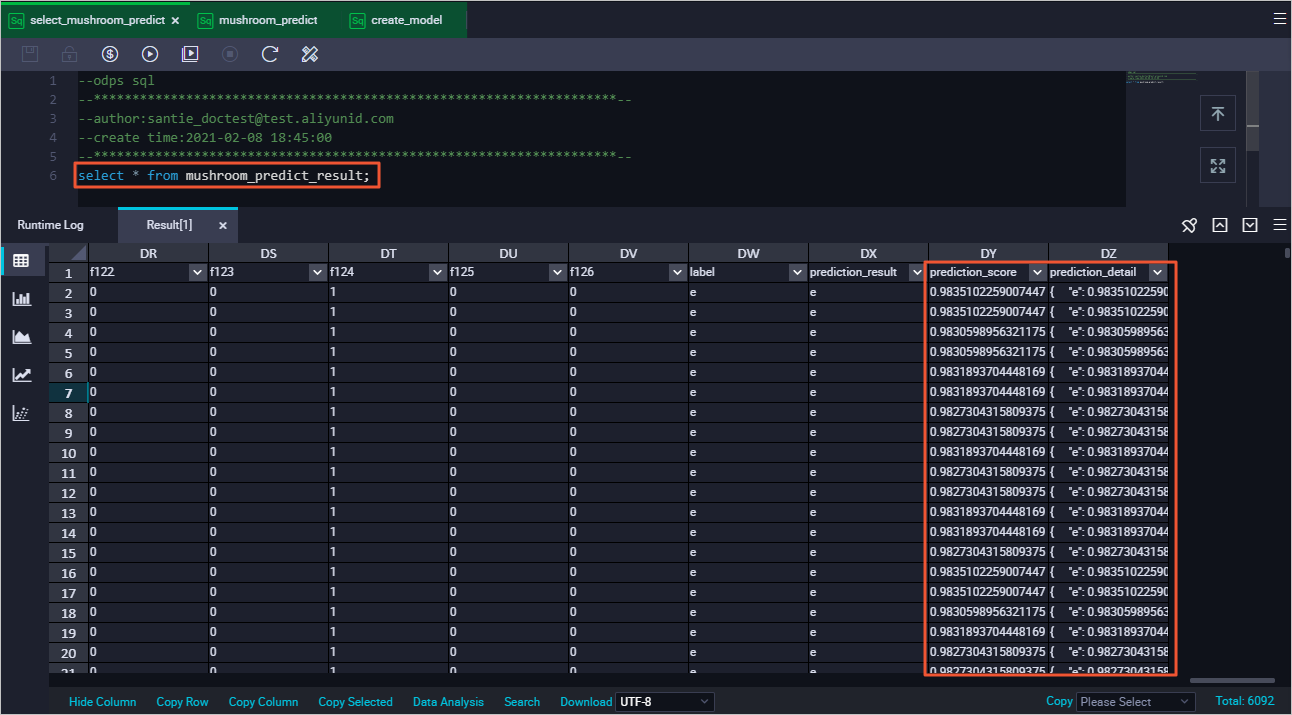This topic provides an example to describe how to use MaxCompute SQLML and the logistic regression for binary classification model of Machine Learning Platform for AI (PAI). In this example, Mushroom Data Set, an open source dataset is used to make predications about whether mushrooms are toxic.

Prerequisites

An Alibaba Cloud account is created and passes the real-name verification. For more information, see Create an Alibaba Cloud account.

If you want to perform operations as a RAM user, make sure that the RAM user is available and authorized. For more information, see Prepare a RAM user.

Procedure

1. Optional:Activate a MaxCompute pay-as-you-go resource package, DataWorks Basic, and Pay-As-You-Go (PAI Studio, DSW, and EAS). The services must be in the same region.
1. Go to the product page of Alibaba Cloud MaxCompute and click Buy Now.
Note
• If you have not activated MaxCompute but use this method to activate MaxCompute, DataWorks Basic and a MaxCompute pay-as-you-go resource package are activated by default.
• If you have activated a MaxCompute pay-as-you-go resource package, skip this step.
2. Go to the buy page of DataWorks and activate DataWorks Basic.
Note If you have activated DataWorks Basic, skip this step.
3. Go to the buy page of PAI and activate Pay-As-You-Go (PAI Studio, DSW, and EAS).
For more information, see Activate PAI.Note If you have activated Pay-As-You-Go (PAI Studio, DSW, and EAS), skip this step.
2. Download the Mushroom Data Set file named agaricus-lepiota.data and save it as a TXT, CSV, or LOG file. Example: agaricus-lepiota.data.txt.
3. Log on to the DataWorks console and create or configure a DataWorks workspace.
• If a DataWorks workspace is available, go to the Workspaces page. Find the workspace that you want to manage and click Modify service configuration in the Actions column. In the panel that appears, set Compute Engines to MaxCompute (pay-as-you-go) and Machine Learning Services to PAI Studio (pay-as-you-go).• If no DataWorks workspaces are available, create one. In the Create Workspace panel, set Compute Engines to MaxCompute (pay-as-you-go) and Machine Learning Services to PAI Studio (pay-as-you-go). For more information, see Create a workspace.4. In the DataWorks console, create a table named mushroom_classification and import the prepared dataset.
1. Find the workspace that you want to manage and click Data Analytics in the Actions column. On the page that appears, create a table named mushroom_classification.

The following DDL statement is used to create a table:

create table mushroom_classification (
label      string               comment 'poisonous=p,edible=e',
cap_shape  string               comment 'bell=b,conical=c,convex=x,flat=f,knobbed=k,sunken=s',
cap_surface string              comment 'fibrous=f,grooves=g,scaly=y,smooth=s',
cap_color string                comment 'brown=n,buff=b,cinnamon=c,gray=g,green=r,pink=p,purple=u,red=e,white=w,yellow=y',
bruises string                  comment 'bruises=t,no=f',
odor string                     comment 'almond=a,anise=l,creosote=c,fishy=y,foul=f,musty=m,none=n,pungent=p,spicy=s',
gill_attachment string          comment 'attached=a,descending=d,free=f,notched=n',
gill_spacing string             comment 'close=c,crowded=w,distant=d',
gill_color string               comment 'black=k,brown=n,buff=b,chocolate=h,gray=g,green=r,orange=o,pink=p,purple=u,red=e,white=w,yellow=y',
stalk_shape string              comment 'enlarging=e,tapering=t',
stalk_root string               comment 'bulbous=b,club=c,cup=u,equal=e,rhizomorphs=z,rooted=r,missing=?',
stalk_surface_above_ring string comment 'fibrous=f,scaly=y,silky=k,smooth=s',
stalk_surface_below_ring string comment 'fibrous=f,scaly=y,silky=k,smooth=s',
stalk_color_above_ring string   comment 'brown=n,buff=b,cinnamon=c,gray=g,orange=o,pink=p,red=e,white=w,yellow=y',
stalk_color_below_ring string   comment 'brown=n,buff=b,cinnamon=c,gray=g,orange=o,pink=p,red=e,white=w,yellow=y',
veil_type string                comment 'partial=p,universal=u',
veil_color string               comment 'brown=n,orange=o,white=w,yellow=y',
ring_number string              comment 'none=n,one=o,two=t',
ring_type string                comment 'cobwebby=c,evanescent=e,flaring=f,large=l,none=n,pendant=p,sheathing=s,zone=z',
spore_print_color string        comment 'black=k,brown=n,buff=b,chocolate=h,green=r,orange=o,purple=u,white=w,yellow=y',
population string               comment 'abundant=a,clustered=c,numerous=n,scattered=s,several=v,solitary=y',
);
2. Import the data in the agaricus-lepiota.data.txt file to the mushroom_classification table. Set Select a method for matching to By Location.
For more information, see Create tables and import data.3. Use the ad hoc query feature of DataWorks, create an ODPS SQL node, and then execute SQL statements to verify the data import results.

Sample statement:
select * from mushroom_classification;
The following results are returned.5. Use one-hot encoding to process data in the mushroom_classification table.
The fields must be numeric for the logistic regression for binary classification model. Therefore, one-hot encoding is used to convert the enumerated values into numeric values. For example, valid values for cap_shape are b, c, x, f, k, and s. One-hot encoding converts the six enumerated values into six columns. Each column corresponds to an enumerated value. If the value of cap_shape is equal to the enumerated value of the column to which it corresponds, enter 1. Otherwise, enter 0.
1. Optional:Create a workflow. Example: mc_test.
Note If you have created a workflow, skip this step.
2. Create an ODPS Script node, write code, use one-hot encoding to process the imported data, and then write the processed data into the new table named mushroom_classification_one_hot.
Sample statement:
create temporary function one_hot as 'onehot.OneHotEncoding' using
#CODE ('lang'='JAVA')
package onehot;

import com.aliyun.odps.udf.UDFException;
import com.aliyun.odps.udf.UDTF;
import com.aliyun.odps.udf.annotation.Resolve;

import java.io.IOException;
import java.util.ArrayList;
import java.util.List;

@Resolve({"string,string,string,string,string,string,string,string,string,string," +
"string,string,string,string,string,string,string,string,string,string,string,string" +
"->" +
"bigint,bigint,bigint,bigint,bigint,bigint,bigint,bigint,bigint,bigint,"+
"bigint,bigint,bigint,bigint,bigint,bigint,bigint,bigint,bigint,bigint," +
"bigint,bigint,bigint,bigint,bigint,bigint,bigint,bigint,bigint,bigint," +
"bigint,bigint,bigint,bigint,bigint,bigint,bigint,bigint,bigint,bigint," +
"bigint,bigint,bigint,bigint,bigint,bigint,bigint,bigint,bigint,bigint," +
"bigint,bigint,bigint,bigint,bigint,bigint,bigint,bigint,bigint,bigint," +
"bigint,bigint,bigint,bigint,bigint,bigint,bigint,bigint,bigint,bigint," +
"bigint,bigint,bigint,bigint,bigint,bigint,bigint,bigint,bigint,bigint," +
"bigint,bigint,bigint,bigint,bigint,bigint,bigint,bigint,bigint,bigint," +
"bigint,bigint,bigint,bigint,bigint,bigint,bigint,bigint,bigint,bigint," +
"bigint,bigint,bigint,bigint,bigint,bigint,bigint,bigint,bigint,bigint," +
"bigint,bigint,bigint,bigint,bigint,bigint,bigint,bigint,bigint,bigint," +
"bigint,bigint,bigint,bigint,bigint,bigint"})
public class OneHotEncoding extends UDTF {
private static char[][] features = {
{ 'b','c','x','f','k','s'}, //cap-shape
{ 'f','g','y','s'}, //cap-surface
{ 'n','b','c','g','r','p','u','e','w','y'}, //cap-color
{ 't','f'}, //bruises
{ 'a','l','c','y','f','m','n','p','s'}, //odor
{ 'a','d','f','n'}, //gill-attachment
{ 'c','w','d'}, //gill-spacing
{ 'b','n'}, //gill-size
{ 'k','n','b','h','g','r','o','p','u','e','w','y'}, //gill-color
{ 'e','t'}, //stalk-shape
{ 'b','c','u','e','z','r','?'}, //stalk-root
{ 'f','y','k','s'}, //stalk-surface-above-ring
{ 'f','y','k','s'}, //stalk-surface-below-ring
{ 'n','b','c','g','o','p','e','w','y'}, //stalk-color-above-ring
{ 'n','b','c','g','o','p','e','w','y'}, //stalk-color-below-ring
{ 'p','u'}, //veil-type
{ 'n','o','w','y'}, //veil-color
{ 'n','o','t'}, //ring-number
{ 'c','e','f','l','n','p','s','z'}, //ring-type
{ 'k','n','b','h','r','o','u','w','y'}, //spore-print-color
{ 'a','c','n','s','v','y'}, //population
{ 'g','l','m','p','u','w','d'}, //habitat
};
@Override
public void process(Object[] objects) throws UDFException, IOException {
List<Long> featuresEncoding = new ArrayList<>(126);
for (int i = 0; i < objects.length; i++) {
String value = (String)objects[i];
char[] feature = features[i];
for (char c : feature) {
featuresEncoding.add(value.charAt(0) == c ? 1L : 0L);
}
}
forward(featuresEncoding.toArray());
}
}

#END CODE;

create table mushroom_classification_one_hot as
select t.*, label
from mushroom_classification
lateral view
one_hot(cap_shape,cap_surface,cap_color,bruises,odor,gill_attachment,
gill_spacing, gill_size, gill_color, stalk_shape,stalk_root ,
stalk_surface_above_ring,stalk_surface_below_ring,stalk_color_above_ring,
stalk_color_below_ring,veil_type,veil_color,ring_number,ring_type,spore_print_color,
population,habitat) t
AS f1,f2,f3,f4,f5,f6,f7,f8,f9,f10,f11,f12,f13,f14,f15,f16,f17,f18,f19,f20,
f21,f22,f23,f24,f25,f26,f27,f28,f29,f30,f31,f32,f33,f34,f35,f36,f37,f38,f39,f40,
f41,f42,f43,f44,f45,f46,f47,f48,f49,f50,f51,f52,f53,f54,f55,f56,f57,f58,f59,f60,
f61,f62,f63,f64,f65,f66,f67,f68,f69,f70,f71,f72,f73,f74,f75,f76,f77,f78,f79,f80,
f81,f82,f83,f84,f85,f86,f87,f88,f89,f90,f91,f92,f93,f94,f95,f96,f97,f98,f99,f100,
f101,f102,f103,f104,f105,f106,f107,f108,f109,f110,f111,f112,f113,f114,f115,f116,
f117,f118,f119,f120,f121,f122,f123,f124,f125,f126;
3. Use the ad hoc query feature of DataWorks, create an ODPS SQL node, and then execute SQL statements to verify the results of one-hot encoding-based processing.
Sample statement:
select * from mushroom_classification_one_hot;
The following results are returned.4. Use the ad hoc query feature of DataWorks, create an ODPS SQL node, and then create a training dataset and a test dataset based on the data in mushroom_classification_one_hot.
Sample statement:
-- Training dataset. About 25% of the data is used to train models.
create table mushroom_training as
select * from mushroom_classification_one_hot where sample(4,1);

-- Test dataset. About the rest 75% of the data is used to predict and evaluate models.
create table mushroom_predict as
select * from mushroom_classification_one_hot except all select * from mushroom_training;
6. Create a machine learning model and make predictions.
1. Use the ad hoc query feature of DataWorks, create an ODPS SQL node, and then create a logistic regression for binary classification model based on the training dataset. The model is named lr_test_model.
Sample statement:
create model lr_test_model
with properties('model_type'='logisticregression_binary', 'goodValue'='p','maxIter'='1000')
as select * from mushroom_training;
Note
• You can specify more parameters in properties. The parameters are the same as those in PAI. For more information, see Logistic Regression for Binary Classification.
• The SQL engine extracts and executes the statement after as and stores the results in a temporary table. You can view the results in Summary in the Logview of a job. The lifecycle of the temporary table is one day. If the table expires, it is automatically deleted.
• If you want to delete the model, execute the drop offlinemodel lr_test_model statement.
2. Use the ad hoc query feature of DataWorks, create an ODPS SQL node, and then use ml_predict to predict the data in the test dataset based on lr_test_model.
Sample statement:
create table mushroom_predict_result as
select * from ml_predict(
lr_test_model,
(select * from mushroom_predict)
);
Note
• The SQL engine saves the subquery results of ml_predict to a temporary table. The lifecycle of the temporary table is one day. If the table expires, it is automatically deleted.
• The results of ml_predict can be placed in the FROM clause of the query statement. You can also execute the INSERT or CREATE TABLE AS statement to save the results to another table. For more information about ml_predict, see Supported model prediction functions.
3. Use the ad hoc query feature of DataWorks, create an ODPS SQL node, and then execute the required statement to view the prediction results for the mushroom_predict_result table.
Sample statement:
select * from mushroom_predict_result;
The following results are returned.7. Use ml_evaluate to evaluate the prediction accuracy of the model.